﻿ 舰载导弹垂直发射时适配器动态特性研究
 舰船科学技术2016, Vol. 38Issue (3): 138-142PDF

1. 海军装备采购中心, 北京 100071;
2. 中国船舶重工集团公司第七一三研究所, 河南郑州 450015

The modeling and mechanical characteristics simulation of adapter in the vertical launching system
ZHANG Qi1, LI Shi-jun2, ZHAO Jian-bo2
1. Equipment Department of PLA Navy, Beijing, 100071;
2. The 713 Research Institute of CSIC, Zhengzhou 450015, China
Abstract: In order to analyze mechanical characteristics of a missile adapter for some vertical missile launching system, aimed at hyperelastic spong material of adapter, mechanical model is got according to experimental data. By using ABAQUS, the nonlinear dynamic model of that vertical launch system which contains adapter is established based on reasonable simplification. The transient dynamics calculation of vertical launching process is carried out by use of central finite difference method and the results reveal the rule of adapter friction and adapter's thickness variation in the process of launching. The structural dynamics model presented here possesses an important reference value and guidance to intensity checkout and structure optimization of adapter.
Key words: adapter    hyperelastic spong    large deformation    vertical launching    structural dynamics
0 引 言

1 超弹性海绵材料本构模型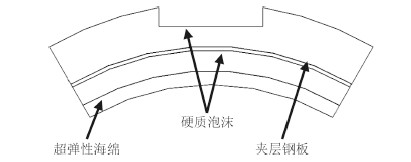图 1 适配器结构 Fig. 1 Schematic diagram of adapter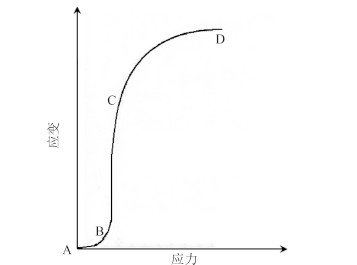图 2 海绵层应力-应变示意图 Fig. 2 Schematic diagram of stress and strain

AB 段（弹性过程）：应变小于5%，主要由海绵材料的空腔壁弯曲引起；

BC 段（屈曲过程）：空腔壁弯曲达到极限时，开始发生屈曲，应力增加一点，而应变增加很多；

CD 段（密实化过程）：由于空腔壁屈曲后变得密实，空腔消失，此时海绵材料所受的压缩主要是海绵材料分子之间的挤压。

 $W = \sum\limits_{i = 1}^N {\frac{{2{\mu _i}}}{{\alpha _i^2}}[\lambda _1^{{\alpha _i}} + \lambda _2^{{\alpha _i}} + \lambda _3^{{\alpha _i}} - 3 + \frac{1}{{{\beta _i}}}({J^{ - {\alpha _i}{\beta _i}}} - 1)]}$ (1)

αiμiβi参数反映了材料的力学特性，需要根据单向试验数据或双向试验数据对应力采用非线性最小二乘法计算。由于这种材料在适配器中只承受径向压缩，因此单向试验数据足够反映该材料的径向力学特性。应变能函数W对于单向压缩试验的伸长率即为工程应变，根据式（1）可得工程应力-应变的关系：

 ${T_U} = \frac{{\partial W}}{{\partial {\lambda _U}}} = \frac{2}{{{\lambda _U}}}\sum\limits_{i = 1}^N {\frac{{{\mu _i}}}{{{\alpha _i}}}\left( {\lambda _U^{{\alpha _i}} - {J^{ - {\alpha _i}{\beta _i}}}} \right)} ,\;1 \le N \le 6。$ (2)

2 舰载导弹垂直发射过程建模 2.1 有限元建模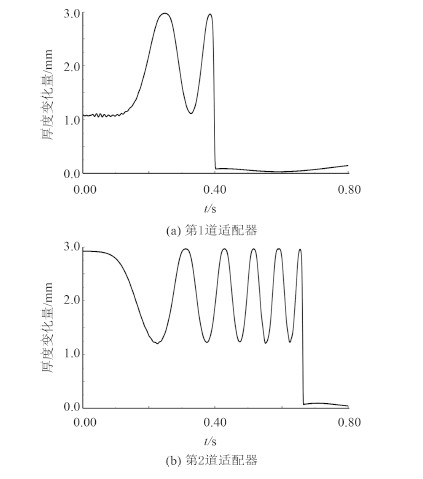图 6 单块适配器厚度变化曲线 Fig. 6 Thickness change of the adapter

2.2 边界条件与载荷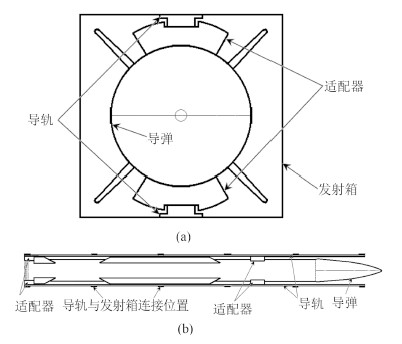图 3 适配器装配位置示意图 Fig. 3 Position of launcher and adapter
2.3 系统结构动力学与计算方法

 $M\left\{ {\ddot u} \right\} + C\left\{ {\dot u} \right\} + K\left\{ u \right\} = \left\{ P \right\}$ (3)

 ${\left\{ {\ddot u} \right\}_t} = {M^{ - 1}}\left( {{P_t} - {I_t}} \right)。$ (4)

 ${I_t} = C{\left\{ {\dot u} \right\}_t} + K{\left\{ u \right\}_t}。$ (5)

 ${\left\{ {\dot u} \right\}_{t + \Delta t}} = {\left\{ {\dot u} \right\}_t} + \frac{{\Delta t}}{2}\left( {{{\left\{ {\ddot u} \right\}}_t} + {{\left\{ {\ddot u} \right\}}_{t + \Delta t}}} \right),$ (6)
 ${\left\{ u \right\}_{t + \Delta t}} = {\left\{ u \right\}_t} + {\left\{ {\dot u} \right\}_t} \cdot \Delta t + \frac{{\Delta {t^2}}}{2}{\left\{ {\ddot u} \right\}_t},$ (7)

 ${\left\{ u \right\}_{t - \Delta t}} = {\left\{ u \right\}_t} - {\left\{ {\dot u} \right\}_t} \cdot \Delta t + \frac{{\Delta {t^2}}}{2}{\left\{ {\ddot u} \right\}_t}。$ (8)

 ${\left\{ {\dot u} \right\}_t} = \frac{{{{\left\{ u \right\}}_{t + \Delta t}} - {{\left\{ u \right\}}_{t - \Delta t}}}}{{2\Delta t}},$ (9)
 ${\left\{ {\ddot u} \right\}_t} = \frac{{{{\left\{ u \right\}}_{t + \Delta t}} - 2{{\left\{ u \right\}}_t} + {{\left\{ u \right\}}_{t - \Delta t}}}}{{\Delta {t^2}}}。$ (10)

 ${\left\{ {\dot u} \right\}_{t + \frac{{\Delta t}}{2}}} = {\left\{ {\dot u} \right\}_{t - \frac{{\Delta t}}{2}}} + \frac{{\left( {\Delta {t_{t + \Delta t}} + \Delta {t_t}} \right)}}{2}{\left\{ {\dot u} \right\}_t},$ (11)
 ${\left\{ u \right\}_{t + \Delta t}} = {\left\{ u \right\}_t} + \Delta {t_{t + \Delta t}}{\left\{ {\dot u} \right\}_{t + \frac{{\Delta t}}{2}}}。$ (12)

3 仿真结果分析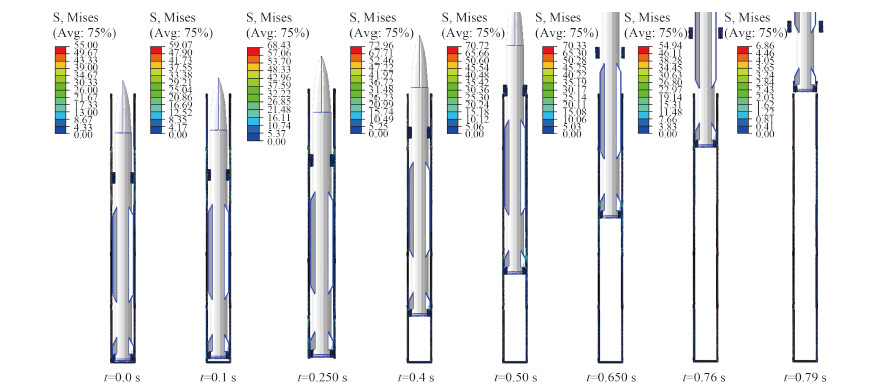图 4 导弹发射过程应力云图 Fig. 4 Adapter stress distribution during missile launching process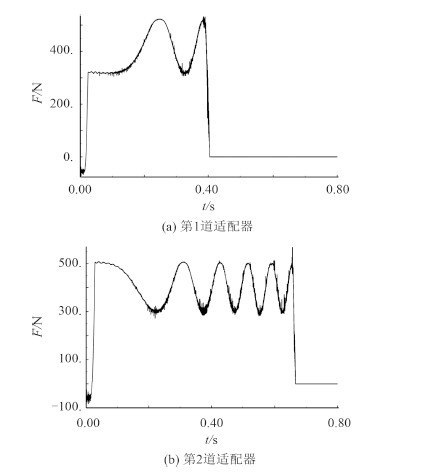图 5 适配器与导轨摩擦力曲线 Fig. 5 Friction force between adapter and lead rail

4 结 语

  王亚锋, 孙富春, 张友安, 等. 一种舰载垂直发射导弹的分段传递对准方法[J]. 系统仿真学报, 2009, 21(2):335-339  尹永鑫, 杨明, 王子才. 并联式运载器的垂直发射建模与控制[J]. 北京航空航天大学学报, 2008, 34(4):369-372, 376.  郑友胜, 王良明. 火箭弹垂直发射动力学建模与仿真[J]. 飞行力学, 2008, 26(2):60-63.  MOORHEAD S B. The latest in ship weapon launchers-the vertical launching system[J]. Naval Engineers Journal, 1981, 93(2):90-96.  YOSHIMOTO B. Vertical launching system (VLS) technical assistance expert system (VTAEXS)[C]//Proceedings of the 11th conference on artificial intelligence for applications. Los Angeles, CA:IEEE, 1995:170-176.  常卫伟. 舰载导弹垂直发射系统综述[J]. 舰载武器, 2002(4):47-52.  郑宏建, 张兴有, 马洪霞. 航空导弹垂直发射系统特点分析[J]. 战术导弹技术, 2002(1):18-22.  徐悦, 张振鹏, 陈小庆. 舰载导弹垂直发射技术研究进展[J]. 导弹与航天运载技术, 2007(3):22-25.  徐悦, 田爱梅, 张振鹏, 等. 导弹垂直发射系统柔性多体动力学建模与仿真[J]. 兵工学报, 2008, 29(9):1083-1087.  朱卫兵, 郜冶. 舰载导弹垂直发射装置模态分析与实验[J]. 哈尔滨工程大学学报, 1998, 19(2):39-44.  庄茁, 由小川, 廖剑晖, 等. 基于ABAQUS的有限元分析和应用[M]. 北京:清华大学出版社, 2009.  BONET J, WOOD R D. Nonlinear continuum mechanics for finite element analysis[M]. Cambridge:Cambridge University Press, 1977.